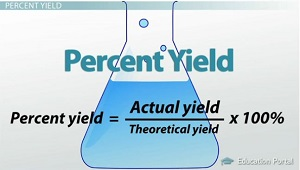# Question fee32

Jan 25, 2014

To calculate the percent yield, you divide the actual yield by the theoretical yield and multiply by 100.

EXAMPLE

What is the percent yield if 0.650 g of copper are formed when excess aluminum reacts with a 2.00 g of copper(II) chloride dihydrate according to the equation

3CuCl₂•2H₂O + 2Al → 3Cu + 2AlCl₃ + 2H₂O

Solution

First, calculate the theoretical yield of Cu.

2.00 g CuCl₂•2H₂O ×

(1 mol CuCl₂•2H₂O)/(170.5 g CuCl₂•2H₂O) × (3 mol Cu)/(3 mol CuCl₂•2H₂O) × (63.55 g Cu)/(1 mol Cu)# =

0.745 g Cu

Now calculate the percent yield.

% yield = (actual yield)/(theoretical yield) × 100 % = $\frac{0.650 g}{0.745 g}$ × 100 % = 87.2 %